# 快速排序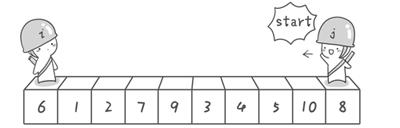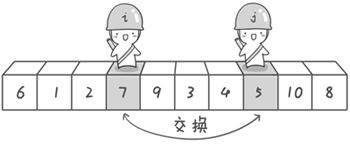6 1 2 5 9 3 4 7 10 8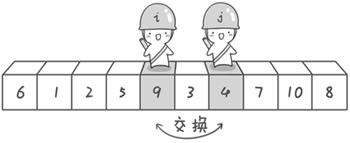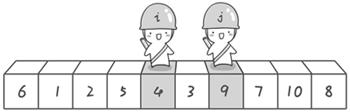6 1 2 5 4 3 9 7 10 8

3 1 2 5 4 6 9 7 10 8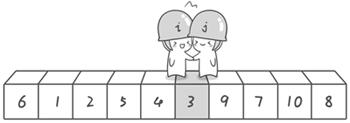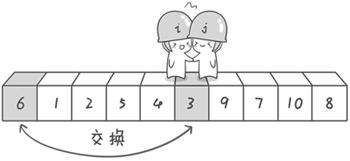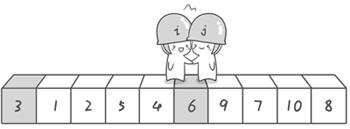OK，解释完毕。现在基准数6已经归位，它正好处在序列的第6位。此时我们已经将原来的序列，以6为分界点拆分成了两个序列，左边的序列是“3 1 2 5 4”，右边的序列是“9 7 10 8”。接下来还需要分别处理这两个序列。因为6左边和右边的序列目前都还是很混乱的。不过不要紧，我们已经掌握了方法，接下来只要模拟刚才的方法分别处理6左边和右边的序列即可。现在先来处理6左边的序列现吧。

1、算法思想：

2、实现方法：

第三步：前两步，完成之后，交换他们的值

3、复杂度分析（最好的请况）

首先：将数组分成log(n)层,，但是每一层都要调用n次 那么则是nlog(n)次 复杂度为O(nlog(n));

4、源代码（ 这里提供算法导论版本的）

#include<iostream>
#include<ctime>
#include<cstdlib>
using namespace std;

void swap(int *a, int *b)
{
int tmp;
tmp = *a;
*a = *b;
*b = tmp;
}
int partion(int a[], int p, int r)
{
int i, j ;
srand((unsigned)time(NULL));
int e = rand()%(r-p+1) + p; //随机生成一个位置 作为哨兵a[e]
swap(&a[e], &a[r]);  //交换末尾位置和哨兵的值 此时 a[r] = a[e]
int key = a[r];  // 右边作为基准 那么从左往右找，左边作为基准，从右往左找
i = p-1; // 最左边的数
for(j = p; j < r; j++)
{// 从左往右找，好到第一个小于基准的数 交换他们的顺序
if(a[j]<=key)
{
swap(&a[i+1],&a[j]);
i++;
}
}
swap(&a[i+1], &a[r]); //把基准放到他该放在的位置 i+1上，i+1这个就是排序排好了的位置
return i+1;
}
void QuickSort(int a[], int p, int r)
{   //这个条件是因为
if(p <r)
{
int q = partion(a, p, r);
QuickSort(a, p, q-1 );
QuickSort(a, q+1,r);
}
}
int main(){
int array[]={0,-2,11,-4,13,-5,14,-43};
QuickSort(array,0,7);
for(int i=0;i<=7;i++)
cout<<array[i]<<" ";
cout<<endl;
return 0;
}

public class QuickSortTest {

/**
* @param args
*/
public static void main(String[] args) {
int a[] = {1,3,2,56,2,3,0};
int k = 3;
//      quickSort(a,0,a.length,3);
quickSort(a,0,a.length-1);
for(int i = 0; i < a.length; i++){
System.out.println(a[i]);
}

}

/**
* @param a
* @param i
* @param length
*/
private static void quickSort(int[] a, int start, int end) {
int left = start;
int right = end;
int temp ;
if(left >right ){
return;
}
temp = a[start];

while(left!=right){
while(left<right && a[right] >=temp){
// 理解循环，能进入的必定是满足while括号里面的条件的
right--;
}

while(left <right && a[left] <=temp){
left++;
}
// 左边找到第一个大于pivo的数字,右边找到第一个小于pivo的数字 交换他们的顺序
if(left <right){
int temp2 = a[left];
a[left] = a[right];
a[right]= temp2;
}
}
// 上面肯定left== right 找到了基准位置应该在的位置
a[start] = a[left];
a[left] = temp;

quickSort(a, start, left-1);
quickSort(a, left+1, end);

}

/**
* @param a
* @param i
* @param length
* @param j
*/
private static void quickSort(int[] a, int start, int end, int j) {
int left = start;
int right = end;

if(left >= right)
{
return;
}
//选择一个哨兵
int temp = a[left];

while(left!=right){
// 先从右边往左边找
while(left<right && a[right] >=temp){
right--;
}
if(left <right){
a[left++] = a[right];
}

//从左边找到一个小于temp的数字，放到右边去
while(left <right && a[left] <=temp){
left++;
}
if(left <right){
a[right--] = a[left];
}
}
a[right]= temp;

//       if(left)

}

}


wangxiaoming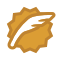CSDN认证博客专家 架构 Spring Boot Redis

03-131537

#### 快速排序法

09-1034万+

#### 快速排序---(面试碰到过好几次)03-053万+

#### 图解快速排序（C++实现）

06-124万+

#### 快速排序算法——C/C++

06-15187

#### 算法-排序那些事

08-034660

#### 最常用的排序——快速排序

07-0222万+

#### 快速排序基本思路（通俗易懂+例子）

08-1362万+

#### 白话经典算法系列之六 快速排序 快速搞定©️2020 CSDN 皮肤主题: Age of Ai 设计师: meimeiellie点击重新获取扫码支付1.余额是钱包充值的虚拟货币，按照1:1的比例进行支付金额的抵扣。
2.余额无法直接购买下载，可以购买VIP、C币套餐、付费专栏及课程。余额充值